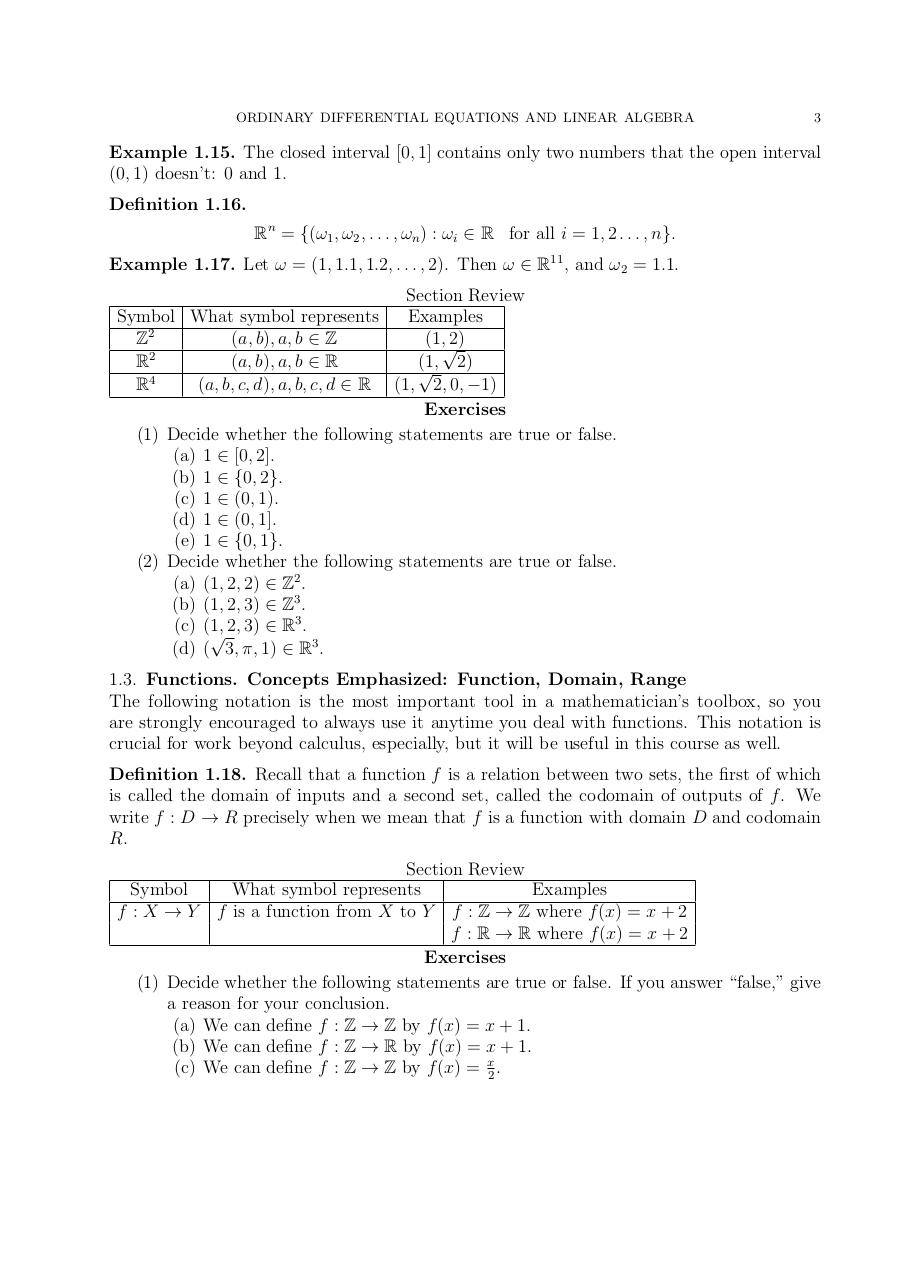# ODELAClahane061416.pdfPage 1 2 34570

#### Text preview

ORDINARY DIFFERENTIAL EQUATIONS AND LINEAR ALGEBRA

3

Example 1.15. The closed interval [0, 1] contains only two numbers that the open interval
(0, 1) doesn’t: 0 and 1.
Definition 1.16.
Rn = {(ω1 , ω2 , . . . , ωn ) : ωi ∈ R for all i = 1, 2 . . . , n}.
Example 1.17. Let ω = (1, 1.1, 1.2, . . . , 2). Then ω ∈ R11 , and ω2 = 1.1.
Section Review
Symbol What symbol represents
Examples
2
Z
(a, b), a, b ∈ Z
(1,√2)
2
R
(a, b), a, b ∈ R
(1, 2)

4
R
(a, b, c, d), a, b, c, d ∈ R (1, 2, 0, −1)
Exercises
(1) Decide whether the following statements are true or false.
(a) 1 ∈ [0, 2].
(b) 1 ∈ {0, 2}.
(c) 1 ∈ (0, 1).
(d) 1 ∈ (0, 1].
(e) 1 ∈ {0, 1}.
(2) Decide whether the following statements are true or false.
(a) (1, 2, 2) ∈ Z2 .
(b) (1, 2, 3) ∈ Z3 .
3
(c) (1,
√ 2, 3) ∈ R . 3
(d) ( 3, π, 1) ∈ R .
1.3. Functions. Concepts Emphasized: Function, Domain, Range
The following notation is the most important tool in a mathematician’s toolbox, so you
are strongly encouraged to always use it anytime you deal with functions. This notation is
crucial for work beyond calculus, especially, but it will be useful in this course as well.
Definition 1.18. Recall that a function f is a relation between two sets, the first of which
is called the domain of inputs and a second set, called the codomain of outputs of f . We
write f : D → R precisely when we mean that f is a function with domain D and codomain
R.
Section Review
Symbol
What symbol represents
Examples
f : X → Y f is a function from X to Y f : Z → Z where f (x) = x + 2
f : R → R where f (x) = x + 2
Exercises
(1) Decide whether the following statements are true or false. If you answer “false,” give